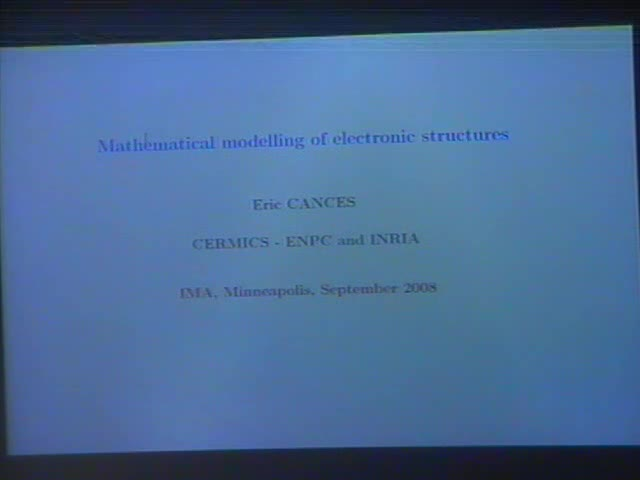Mathematical modeling of electronic structures

September 26, 2008
IMA
Presenters: Eric Cances

Watch Video

Abstract

Quantum Chemistry aims at understanding the properties of matter through the modelling of its behaviour at a subatomic scale, where matter is described as an assembly of nuclei and electrons.

At this scale, the equation that rules the interactions between these constitutive elements is the Schrdinger equation. It can be considered (except in few special cases notably those involving relativistic phenomena or nuclear reactions) as a universal model for at least three reasons. First it contains all the physical information of the system under consideration so that any of the properties of this system can be deduced in theory from the Schrdinger equation associated to it. Second, the Schrdinger equation does not involve any empirical parameter, except some fundamental constants of Physics (the Planck constant, the mass and charge of the electron, ...); it can thus be written for any kind of molecular system provided its chemical composition, in terms of natures of nuclei and number of electrons, is known. Third, this model enjoys remarkable predictive capabilities, as confirmed by comparisons with a large amount of experimental data of various types.

Unfortunately, the Schrödinger equation cannot be directly simulated, except for very small chemical systems. It indeed reads as a time-dependent 3(M+N)-dimensional partial differential equation, where M is the number of nuclei and N the number of the electrons in the system under consideration. On the basis of asymptotic and semiclassical limit arguments, it is however often possible to approximate the Schrdinger dynamics by the so-called Born-Oppenheimer dynamics, in which nuclei behave as classical point-like particles. The internuclei (or interatomic) potential can be computed ab initio, by solving the time-independent electronic Schrödinger equation.

The latter equation is a 3N-dimensional partial differential equation (it is in fact a spectral problem), for which several approximation methods are available. The main of them are the wavefunction methods and the Density Functional Theory (DFT).

In my first lecture (Mathematical modelling of electronic structures), I will present the mathematical properties of the time-independent electronic Schrödinger equation, and show how to construct variational approximations of this equation, in the framework of wavefunction methods. I will mainly deal with the Hartree-Fock approximation; more advanced wavefunction methods will then be presented in the lectures by L. Slipchenko and A. Krylov.

In my second lecture (Mathematical aspects of density functional theory), I will examine the mathematical foundations of DFT. I will compare the constrained-search approach proposed by Levy and involving pure states, with the one proposed by Lieb and involving mixed states. These two approaches lead to Kohn-Sham and extended Kohn-Sham models respectively. I will then review the mathematical properties of the Kohn-Sham LDA and GGA models (corresponding to the first two rungs of the ladder of approximations previously presented by J. Perdew). Lastly, I will introduce the concept of bulk (or thermodynamic) limit, which allows one to rigorously derive DFT models for the condensed phase from molecular DFT models by letting the number of nuclei and electrons go to infinity in an appropriate way.